##账号 自动登录 找回密码 密码 立即注册
 搜索

# [已解决]【C++板块提升计划】梦想护卫舰 第13期 细胞分裂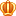发表于 4 天前 | 显示全部楼层 |阅读模式

### 马上注册，结交更多好友，享用更多功能^_^

x

Hanks 博士是 BT（Bio-Tech，生物技术）领域的知名专家。现在，他正在为一个细胞实验做准备工作：培养细胞样本。

Hanks 博士手里现在有 N 种细胞，编号从 1～N，一个第 i 种细胞经过 1 秒钟可以分裂为 S
i 个同种细胞（Si为正整数）。现在他需要选取某种细胞的一个放进培养皿，让其自由分裂，进行培养。一段时间以后，再把培养皿中的所有细胞平均分入 M 个试管，形成 M 份样本，用于实验。Hanks 博士的试管数 M很大，普通的计算机的基本数据类型无法存储这样大的 M 值，但万幸的是，MM 总可以表示为 m1的m2 次方， 其中 m1 ,m 2均为基本数据类型可以存储的正整数。

1. 1
2. 2 1
3. 3

1. -1

1. 2
2. 24 1
3. 30 12

1. 2

【输入输出样例 1 说明】

【输入输出样例 2 说明】

[/hide]

（你们积极参与我就有机会申请精华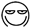3 天前
sfqxx 发表于 2023-1-26 17:12

。。那你还是用这个吧，我刚有个特殊判定没加上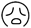1. kinds = input()
2. m1,m2 = input().split()
3. splits = input().split()
4. m1 = int(m1)
5. m2 = int(m2)
6. time = set()
7. pri_m = set()
8. pri_tm = set()

9. for factor in range(2,m1+1):
10.     if m1 % factor ==0:
12.         for f in pri_m:
13.             if factor % f == 0:
15.                 break
17.             tm = 0
18.             while m1 % factor == 0:
19.                 m1 /= factor
20.                 tm += 1

23. maxtm = max(pri_tm)*m2 if pri_tm else 0
24. for sp in splits:
25.     sp = int(sp)
26.     if len([sp for m in pri_m if sp % m == 0]) == len(pri_m):
27.         pri_ts = set()
28.
29.         for t in pri_m:
30.             ts = 0
31.             while sp % t == 0:
32.                 sp /= t
33.                 ts += 1
34.
36.
37.         maxts= max(pri_ts) if pri_ts else 1

38.         if maxtm % maxts == 0:
40.         else:
41.             for t in range(2,maxtm+1):
42.                 if maxts *t > maxtm:
44.                     break
45.     else:
47.
49. if time:
50.     print(min(time))
51. else:
52.     print(-1)

### 评分zhangjinxuan + 5 + 5 鱼C有你更精彩^_^liuhongrun2022 + 2 + 2 + 3

### 本帖被以下淘专辑推荐:发表于 4 天前 | 显示全部楼层
 抱歉了，我数学不好。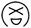这题太难 先不论这题如何解决，单是计算试管有几个都成问题，试问如何算出 30000^10000 是多少呢？另外分裂 S 可以最多是 2×10 的9次方（这只是第一个时间单位），第二个时间单位再分裂的话根本没有办法算了吧？接近无限 infinity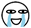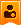楼主| 发表于 4 天前 | 显示全部楼层
 傻眼貓咪 发表于 2023-1-25 17:27 抱歉了，我数学不好。 这题太难 先不论这题如何解决，单是计算试管有几个都成问题，试问如何算 ... 您点击原创链接看看，我复制来可能会有细微差别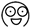发表于 4 天前 | 显示全部楼层
 sfqxx 发表于 2023-1-25 17:28 您点击原创链接看看，我复制来可能会有细微差别 看了，哈哈哈，我还是不会，数据太大了发表于 4 天前 | 显示全部楼层
 我提供个思路：（不知道正不正确） 分解质因数 24=2^3*3; 12= 2^2*3 ;12^2 =2^4*3^2可以包含24((2^3*3)*2*3) 30= 2*3*5;30^3=2^3*3^3*5^3可以包含24((2^3*3)*3^2*5^3)楼主| 发表于 4 天前 | 显示全部楼层
 jhq999 发表于 2023-1-25 17:41 我提供个思路：（不知道正不正确） 分解质因数 24=2^3*3; 对的，我忘记说了楼主| 发表于 4 天前 | 显示全部楼层
 傻眼貓咪 发表于 2023-1-25 17:33 看了，哈哈哈，我还是不会，数据太大了 去参加一下我的鱼币活动呗，顺便测测运气发表于 4 天前 | 显示全部楼层
 密码已经被破译了，小甲鱼都被救出来了发表于 4 天前 | 显示全部楼层
 傻眼貓咪 发表于 2023-1-25 17:27 抱歉了，我数学不好。 这题太难 先不论这题如何解决，单是计算试管有几个都成问题，试问如何算 ... 分 解 只 因 数发表于 4 天前 | 显示全部楼层
 额，标题改一下，最后的密码破译改成细胞分裂发表于 4 天前 | 显示全部楼层发表于 4 天前 | 显示全部楼层
 #这里提供一下C++的答案 #include using namespace std; int n, m1, m2;复制代码 第二行，C++ 的注释是    // 开头的楼主| 发表于 4 天前 From FishC Mobile | 显示全部楼层
 zhangjinxuan 发表于 2023-1-25 20:14 额，标题改一下，最后的密码破译改成细胞分裂 啊呀，尴尬极了发表于 4 天前 | 显示全部楼层
 数学要学好哇~（我**干嘛要选数学题楼主| 发表于 4 天前 From FishC Mobile | 显示全部楼层
 zhangjinxuan 发表于 2023-1-25 22:20 数学要学好哇~（我**干嘛要选数学题 跟数学没关系吧发表于 3 天前 | 显示全部楼层
 sfqxx 发表于 2023-1-25 22:39 跟数学没关系吧 肯定有啊，分解质因数难道不是数学吗？发表于 3 天前 | 显示全部楼层 想知道小甲鱼最近在做啥？请访问 -> ilovefishc.com 回复 支持 反对发表于 3 天前 | 显示全部楼层
 我盯了一下午，用Python写出来，顺便在洛谷看看他们怎么写的，结果全是用C写的kinds = input() m1,m2 = input().split() m1 = int(m1) m2 = int(m2) splits = input().split() time = set() pri_m = set() pri_tm = set() for factor in range(2,m1+1):     if m1 % factor ==0:         ifadd = True         for f in pri_m:             if factor % f == 0:                 ifadd = False                 break         if ifadd:             tm = 0             while m1 % factor == 0:                 print(f"m1:     {m1}/{factor}={m1/factor},{tm}+1")                 m1 /= factor                 tm += 1             pri_tm.add(tm)             pri_m.add(factor)   maxtm = max(pri_tm)*m2 print(pri_m,pri_tm,maxtm) for sp in splits:     print(f"___{sp}____")     sp = int(sp)     if len([sp for m in pri_m if sp % m == 0]) == len(pri_m):         print(sp)         pri_ts = set()                  for t in pri_m:             ts = 0             while sp % t == 0:                 print(f"splits:{sp}/{t}={sp/t},{ts}+1")                 sp /= t                 ts += 1                             pri_ts.add(ts)                      maxts= max(pri_ts)            print(pri_ts,pri_tm)         if maxtm % maxts == 0:             time.add(maxtm // maxts)         else:             for t in range(2,maxtm+1):                 if maxts *t > maxtm:                     time.add(t)                     break         print(time)     else:         time.add(-1)              time.discard(-1) if time:     print(min(time)) else:     print(-1)                           复制代码 改了好多遍了，也不想写注释，就这样吧 写下来最大的感想就是起名字好难啊发表于 3 天前 | 显示全部楼层
 等会，我刚交的是观赏版kinds = input() m1,m2 = input().split() m1 = int(m1) m2 = int(m2) splits = input().split() time = set() pri_m = set() pri_tm = set() for factor in range(2,m1+1):     if m1 % factor ==0:         ifadd = True         for f in pri_m:             if factor % f == 0:                 ifadd = False                 break         if ifadd:             tm = 0             while m1 % factor == 0:                 m1 /= factor                 tm += 1             pri_tm.add(tm)             pri_m.add(factor)   maxtm = max(pri_tm)*m2 for sp in splits:     sp = int(sp)     if len([sp for m in pri_m if sp % m == 0]) == len(pri_m):         pri_ts = set()                  for t in pri_m:             ts = 0             while sp % t == 0:                 sp /= t                 ts += 1                             pri_ts.add(ts)                      maxts= max(pri_ts)            if maxtm % maxts == 0:             time.add(maxtm // maxts)         else:             for t in range(2,maxtm+1):                 if maxts *t > maxtm:                     time.add(t)                     break     else:         time.add(-1)              time.discard(-1) if time:     print(min(time)) else:     print(-1)                           复制代码 (不要问观赏版是什么，问就改bug顺便问问你们是怎么改bug的)发表于 3 天前 | 显示全部楼层
 。。要不要我再加一下特判您需要登录后才可以回帖 登录 | 立即注册 本版积分规则 回帖后跳转到最后一页

GMT+8, 2023-1-29 18:04